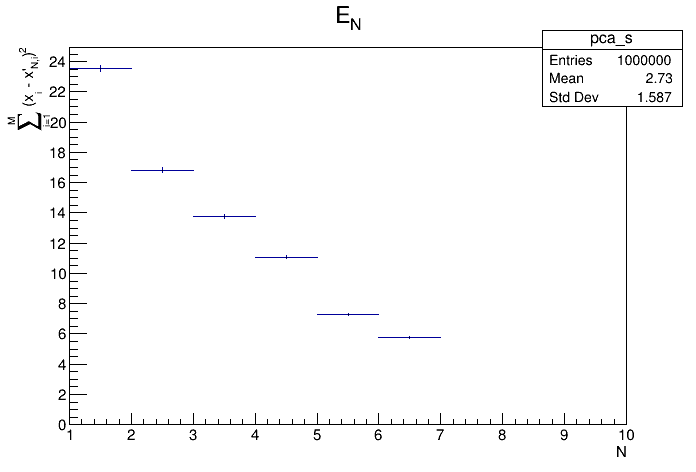# principal¶

Principal Components Analysis (PCA) example

Example of using TPrincipal as a stand alone class.

I create n-dimensional data points, where c = trunc(n / 5) + 1 are correlated with the rest n - c randomly distributed variables.

Based on principal.C by Rene Brun and Christian Holm Christensen

Author: Juan Fernando, Jaramillo Botero
This notebook tutorial was automatically generated with ROOTBOOK-izer from the macro found in the ROOT repository on Sunday, October 02, 2022 at 09:32 AM.

In :
from ROOT import TPrincipal, gRandom, TBrowser, vector

n = 10
m = 10000

c = int(n / 5) + 1

print ("""*************************************************
*         Principal Component Analysis          *
*                                               *
*  Number of variables:           {0:4d}          *
*  Number of data points:         {1:8d}      *
*  Number of dependent variables: {2:4d}          *
*                                               *
*************************************************""".format(n, m, c))

Welcome to JupyROOT 6.27/01
*************************************************
*         Principal Component Analysis          *
*                                               *
*  Number of variables:             10          *
*  Number of data points:            10000      *
*  Number of dependent variables:    3          *
*                                               *
*************************************************


Initilase the TPrincipal object. Use the empty string for the final argument, if you don't wan't the covariance matrix. Normalising the covariance matrix is a good idea if your variables have different orders of magnitude.

In :
principal = TPrincipal(n, "ND")


Use a pseudo-random number generator

In :
randumNum = gRandom


Make the m data-points Make a variable to hold our data Allocate memory for the data point

In :
data = vector('double')()
for i in range(m):
# First we create the un-correlated, random variables, according
# to one of three distributions
for j in range(n - c):
if j % 3 == 0:
data.push_back(randumNum.Gaus(5, 1))
elif j % 3 == 1:
data.push_back(randumNum.Poisson(8))
else:
data.push_back(randumNum.Exp(2))

# Then we create the correlated variables
for j in range(c):
data.push_back(0)
for k in range(n - c - j):
data[n - c + j] += data[k]

# Finally we're ready to add this datapoint to the PCA
data.clear()


Do the actual analysis

In :
principal.MakePrincipals()


Print out the result on

In :
principal.Print()

 Variable #  | Mean Value |   Sigma    | Eigenvalue
-------------+------------+------------+------------
0 |      4.994 |     0.9926 |     0.3856
1 |      8.011 |      2.824 |      0.112
2 |      2.017 |      1.992 |     0.1031
3 |      4.998 |     0.9952 |     0.1022
4 |      8.019 |      2.794 |    0.09998
5 |      1.976 |      2.009 |     0.0992
6 |      4.996 |     0.9996 |    0.09794
7 |      35.01 |      5.147 |  1.409e-16
8 |      30.01 |      5.041 |  2.723e-16
9 |      28.04 |      4.644 |  4.578e-16



Test the PCA

In :
principal.Test()

Info in <TCanvas::MakeDefCanvas>:  created default TCanvas with name c1


Make some histograms of the orginal, principal, residue, etc data

In :
principal.MakeHistograms()


Make two functions to map between feature and pattern space Start a browser, so that we may browse the histograms generated above

In :
principal.MakeCode()
b = TBrowser("principalBrowser", principal)

Writing on file "pca.C" ... done

Warning in <TBrowser::TBrowser>: The ROOT browser cannot run in batch mode


Draw all canvases

In :
from ROOT import gROOT
gROOT.GetListOfCanvases().Draw()